# Termination of the sixth Goodstein Sequence

 Importance: Low ✭
 Author(s): Graham, Ronald L.
 Subject: Logic
 Keywords: Goodstein Sequence
 Prize: \$25 Graham
 Posted by: mdevos on: October 7th, 2008
Question   How many steps does it take the sixth Goodstein sequence to terminate?

For a positive integer, theGoodstein Sequence is defined as follows. The first term of the sequence in. To obtain theterm, write theterm in hereditary base k notation, change all's to's and then subtract 1. If the sequence hits 0, then it terminates. So, the first terms of the sixth Goodstein Sequence are as follows:Surprisingly, despite the fact that Goodstein Sequences grow quite quickly at the start, all such sequences do eventually hit 0 and terminate. This result, first discovered by Goodstein, is of interest in logic since it cannot be proved in Peano arithmetic.

Although determining particular properties of a specific Goodstein Sequence are of limited mathematical value, this problem is an interesting computational challenge.

## Bibliography

* indicates original appearance(s) of problem.

### approximate value

So how much isin terms of, say, Knuth arrows? we haveThat's about as close an approximation as you can get.

### The actual value is much higher

You've underestimated the true value by quite bit.

To get the value of the Goodstein function at n, you take n, write it in hereditary base 2, then replace every appearance to with the infinite ordinal. Call the result R(n). The value of G(n) is then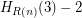whereis the Hardy hierarchy, defined by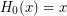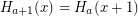for limit ordinals a

So to find G(6), we write, so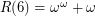. Hence,whereis the fast-growing hierarchy, defined by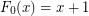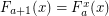for limit ordinals a

(or you could just leave the answer in terms of the Hardy hierarchy, I just changed to the fast-growing hierarchy because the answer is a little simpler.)

### Solutionamount of steps to reduceto -1If you do this a - 1 times:Which means it is reduced to a 0th power and will takesteps to finish.

So total steps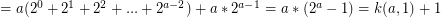For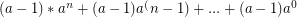: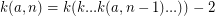[k a times]Where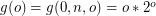,[n copies of g] andis the first number in the sequence in the formE.g.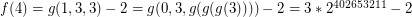Using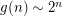:### Has this solution been

Has this solution been verified by the author? Just curious.

### Nah, I just posted it here

Nah, I just posted it here as my attempt at a solution. It probably needs to be done a bit better, but I believe it works until n = 8 or so, where you have to do a bit extra. I might try make it a bit clearer and rigorous at some point. Plus a better approximation would be useful.